# Moms math for windows

This software teaches the basics of addition, subtraction, multiplication, division, fractions, and algebra. Each math category has four levels of instruction, beginning with the beginner level. For the beginners level, objects are shown on the screen to demonstrate the concepts. For example, for subtraction of three from nine, nine objects are shown in red and three of those are in black to demonstrate that three red objects are taken away. Your child can count the number of red objects to get the answer. Similar techniques are used for the other math categories. For example, when multiplying three and four, three columns and four rows of objects are shown. Your child can count the objects to find the answer.

This software was written by Dr. Andrew Motes who wrote the award-winning software, School-Mom, in the late 1980s for MS-DOS PCs. Later, the math instruction was extracted and published as Moms Math for MS-DOS PCs. Now, Dr. Motes has resurrected Moms Math and published it as a W

## Related software (5)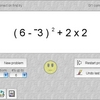## Smooth Operators

Smooth Operators is a complete solution for learning, practicing, and testing the order of operations. An interactive lesson teaches concepts. Practice by clicking operands, or tes ...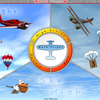## Math Flight 2

Learning mathematics can be a challenge for anyone. Math Flight can help you master it with three fun activities to choose from! With lots of graphics and sound effects, your inter ...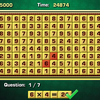## Arithmetic Game

Complete the equations in the interesting arithmetic test!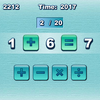## Quick Math

How quickly can you complete the equations with arithmetic symbols?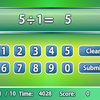## Quick Calculate

Showcase your arithmetic skills to get the answers!